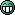# Sequence

#### Danger Phoenix

Soldato
2, 3, 7, 17, 39, ??

a) 58
b) 85
c) 65
d) 75

Also, how do you work out the answer?Soldato
1,234!

Man of Honour
666

Permabanned
80087355

Soldato

#### Jono8

Caporegime
85

I think

the differences are 1,4,10,22,46.

get this by multiplying the difference before by 2 and adding 2.

#### Sinque

Soldato
I just got the same answer, I think.

#### geuben

Associate
I also say 85. The difference of the differences doubles every time.

#### Dharok

Associate
Not sure what the answer is but I know for sure this is a part of the methodology behind it all.Could it be...?!

Last edited:

Associate
5318008!?

Associate
85.

#### Haircut

Soldato
2, 3, 7, 17, 39, ??

2 -> 3 is multiplied by 2, subtract 1
3 -> 7 is multiplied by 2, add 1
7 -> 17 is multiplied by 2, add 3
17 -> 39 is multiplied by 2, add 5
so next one will be multiplied by 2, add 7 which is 85 as people have pointed out.

#### Jono8

Caporegime
2, 3, 7, 17, 39, ??

2 -> 3 is multiplied by 2, subtract 1
3 -> 7 is multiplied by 2, add 1
7 -> 17 is multiplied by 2, add 3
17 -> 39 is multiplied by 2, add 5
so next one will be multiplied by 2, add 7 which is 85 as people have pointed out.

i dont think thats the correct explanation though as the first one doesnt fit.

#### geuben

Associate
i dont think thats the correct explanation though as the first one doesnt fit.

It does if you change subtract 1 to add -1

#### Jono8

Caporegime
It does if you change subtract 1 to add -1

......thats not how these things generally work.

#### geuben

Associate
......thats not how these things generally work.

....and why not?

you add 2 more than the added to the previous number starting from -1

#### Haircut

Soldato
i dont think thats the correct explanation though as the first one doesnt fit.

Of course it fits.

Xn+1 = 2 * Xn + 2(n-0.5) where X0 = 2

if you want to define it like that instead?

#### pingwing

Soldato
85 easy

just need some decent drawing skills to show you the (simple) alevel maths#### Jono8

Caporegime
Of course it fits.

Xn+1 = 2 * Xn + 2(n-0.5) where X0 = 2

if you want to define it like that instead?

Yeh that makes more sense.

#### Jono8

Caporegime
....and why not?

you add 2 more than the added to the previous number starting from -1

i agree. it was the 'subtract' 1 that sounded stupid in my head lol